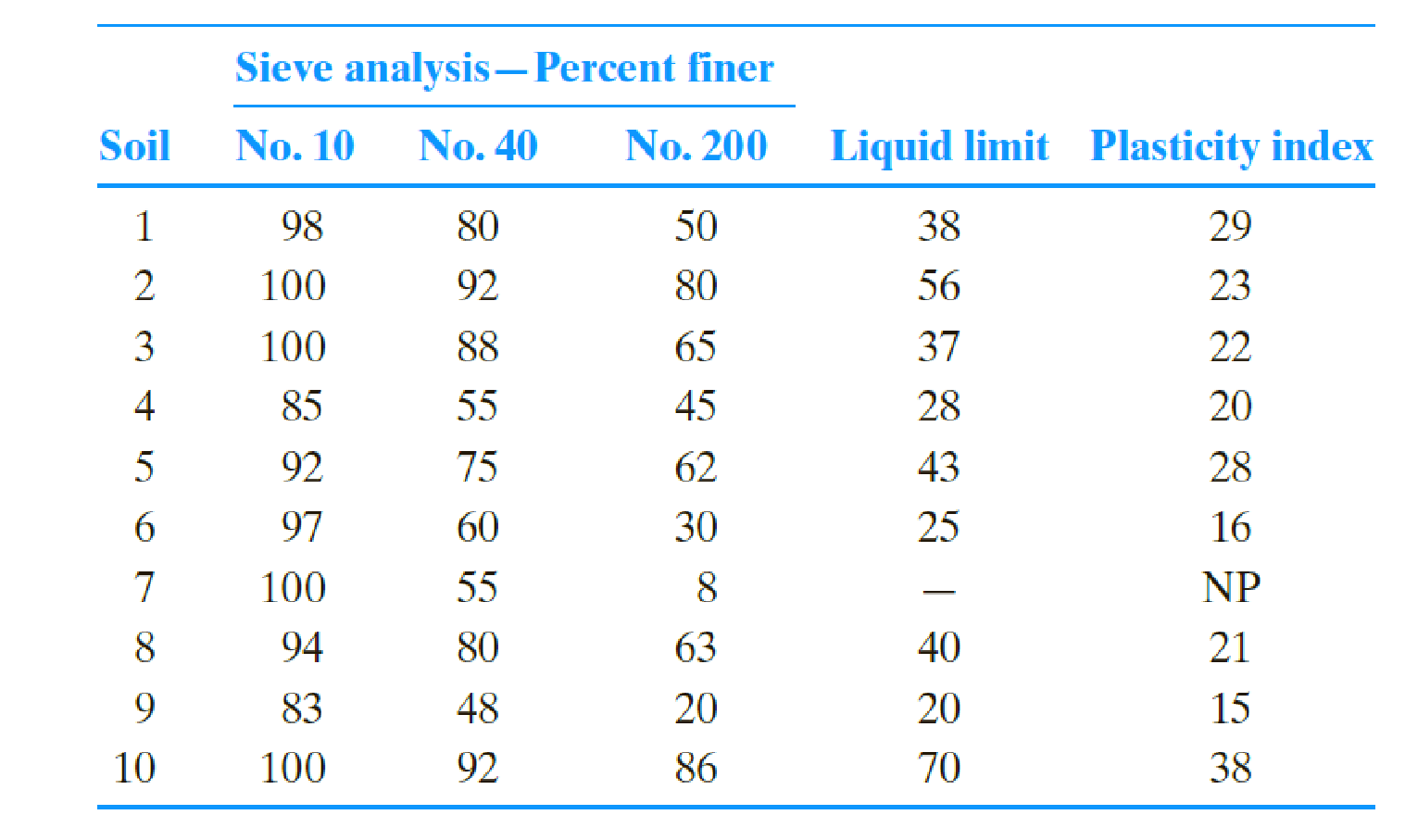Chapter 5, Problem 5.5PPrinciples of Geotechnical Enginee...

9th Edition
Braja M. Das + 1 other
ISBN: 9781305970939

Solutions

Chapter
SectionPrinciples of Geotechnical Enginee...

9th Edition
Braja M. Das + 1 other
ISBN: 9781305970939
Textbook Problem

Determine the group symbols for the fine-grained soils given in Problem 5.4 by the Unified soil classification system.5.4 The sieve analysis of ten soils and the liquid and plastic limits of the fraction passing through the No. 40 sieve are given below. Classify the soils by the AASHTO classification system and give the group index for each soil.To determine

Find the group symbols for the fine-grained soils using the Unified soil classification system.

Explanation

Calculation:

Refer Table (5.2), “Unified soil classification system” in the textbook.

According to Table (5.2), soils 1, 2, 3, 5, 8, and 10 are fine grained soils, because their fine fraction (percent passing No. 200 sieve) is greater than 50 %.

Soil 1:

Refer Figure (5.3), “Plasticity chart” in the textbook.

Mark the plasticity index (29 %) and liquid limit (38 %) in the graph, intersection of the lines takes place above U-line.

Refer Table (5.2), “Unified soil classification system” in the textbook.

The plasticity index (29 %) is greater than 7 and plots on or above “A” mine.

Therefore, name the group symbol of the soil as CL.

Thus, the group symbol of soil 1 using the Unified soil classification system is CL_.

Soil 2:

Refer Figure (5.3), “Plasticity chart” in the textbook.

Mark the plasticity index (23 %) and liquid limit (56 %) in the graph, intersection of the lines takes place below “A” line.

Refer Table (5.2), “Unified soil classification system” in the textbook.

The PI plots below “A” line.

Therefore, name the group symbol of the soil as MH.

Thus, the group symbol of soil 2 using the Unified soil classification system is MH_.

Soil 3:

Refer Figure (5.3), “Plasticity chart” in the textbook.

Mark the plasticity index (22 %) and liquid limit (37 %) in the graph, intersection of the lines takes place below “A” line.

Refer Table (5.2), “Unified soil classification system” in the textbook.

The plasticity index (29 %) is greater than 7 and plots on or above “A” mine.

Therefore, name the group symbol of the soil as CL.

Thus, the group symbol of soil 3 using the Unified soil classification system is CL_

Still sussing out bartleby?

Check out a sample textbook solution.

See a sample solution

The Solution to Your Study Problems

Bartleby provides explanations to thousands of textbook problems written by our experts, many with advanced degrees!

Get Started

What is the difference between work and energy?

Engineering Fundamentals: An Introduction to Engineering (MindTap Course List)

Why is an object said to have greater semantic content than an entity?

Database Systems: Design, Implementation, & Management

What is the purpose of the drill press?

Precision Machining Technology (MindTap Course List)